# [PYTHON] Training data and test data (What are X_train and y_train?) ①

This time in supervised learning Describes training data and test data.

## ■ Training data and test data

Training data: Data that creates a model formula (a formula that expresses the relevance of data) Test data: Data to check how accurate the created formula is

Even if you can express the relevance of data by an expression, it would be a problem if you don't have the data to try it.

Now, let me explain with a simple concrete example.

For example, if you have the following data: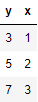Now, divide the data in this table as follows:

Training data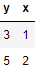test data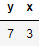At this time, I want to find the relevance of the data first. Consider candidates for y and x formulas (model formulas) from the training data.

Candidate 1: \$ y = 3x \$ Candidate 2: \$ y = 2x + 1 \$

I created a model formula using the training data. However, this alone does not tell us whether this formula really holds. That's why we have test data for verification.

Check if the following data holds for the created model formula. The way to check is to substitute x in the test data into the model formula and see if it really is y.Candidate 1: \$ y = 3x = 3 \ times3 = 9 \$ Candidate 2: \$ y = 2x + 1 = 2 \ times3 + 1 = 7 \$ Correct answer: \$ y = 7 \$

For this reason, regarding the data prepared this time, It turns out that Candidate 2 is more accurate than Candidate 1.

In this way, in machine learning, finding data relevance from training data (creating a model formula) We have a mechanism to check whether the test data is actually accurate.

## ■ Apply X_train and y_train

So what exactly are X_train, y_train, X_test, y_test?

train: Training data (abbreviation of training) test: Test data (data that verifies the model formula)

When applied to the data prepared this time, it looks like this.

Training data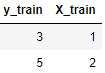test data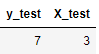As before, consider the model formula from the training data.

Candidate 1: \$ y = 3x \$ Candidate 2: \$ y = 2x + 1 \$

This completes the model formula. After that, we will use test data to verify how accurate these are.

Earlier, substitute x of the test data into the model formula to get the predicted value y. I verified that it matches the actual y.

The predicted value at this time is expressed as y_pred. (Predict)

test dataCandidate 1: \$ y_ {pred} = 3x_ {test} = 3 \ times3 = 9 \$ Candidate 2: \$ y_ {pred} = 2x_ {test} + 1 = 2 \ times3 + 1 = 7 \$ Correct answer: \$ y_ {test} = 7 \$

Therefore, in the model formula created this time It turns out that Candidate 2 is more accurate.

In other words, it is summarized below.

X_train, y_train: Data for creating model formulas (expressions of data relevance) X_test: Data for assigning to the model expression and giving your own answer y_pred y_test: True correct answer data (same as the model answer in mathematics), for answering with your own y_pred

It's a little confusing that only y_test is treated as a model answer, isn't it?

In supervised learning (with test data), basically based on this idea We are creating and verifying various model formulas.